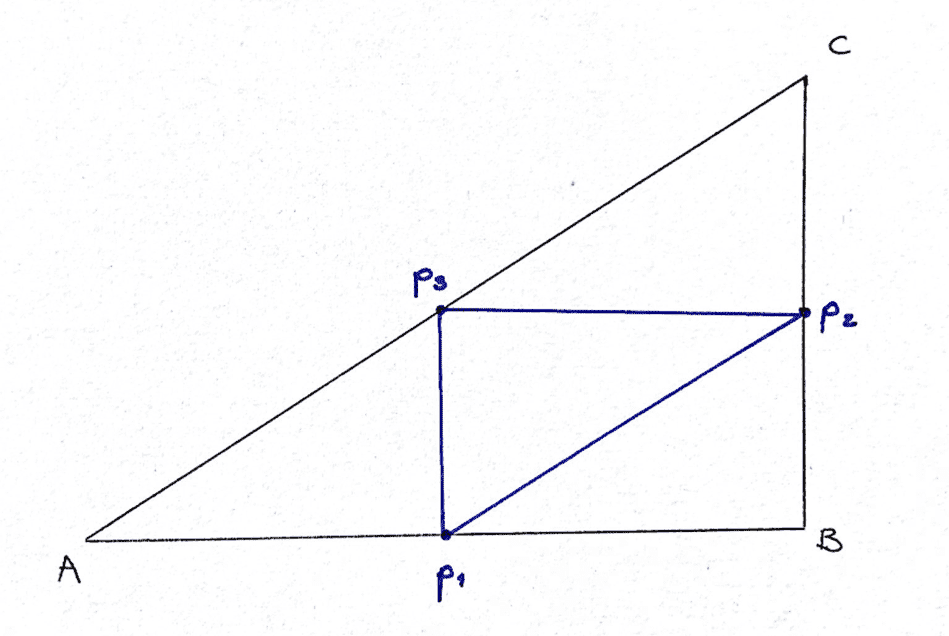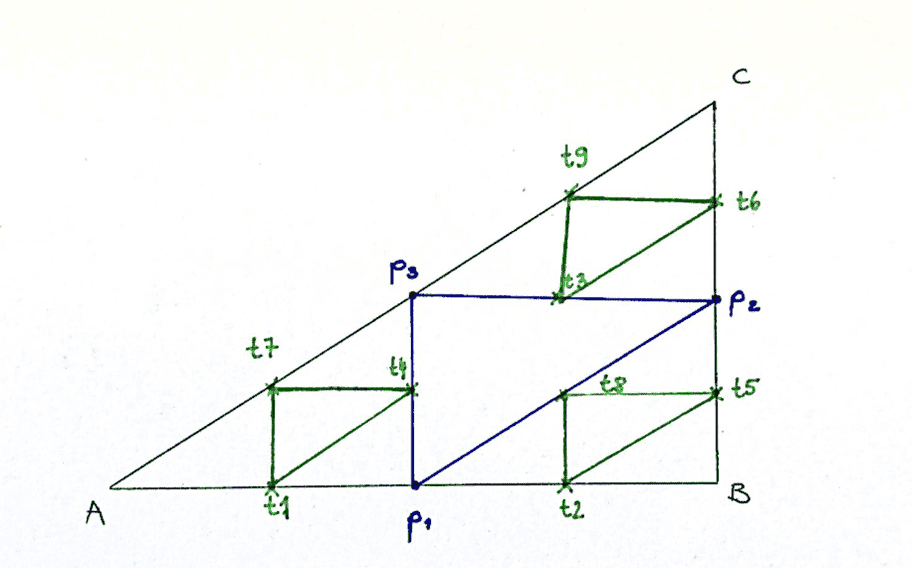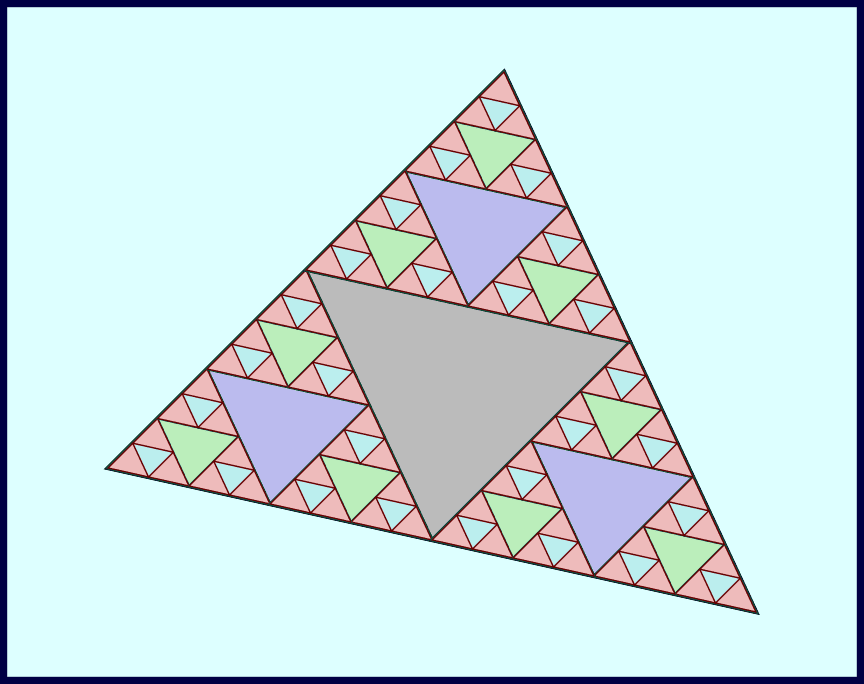# How to write basic syntax for triangle recursion?

bolzano95
TL;DR Summary
I'm trying to program a Sierpinski triangle for n-iterations.
Hi,
I'm new to programming in python [total beginner in programming] and I would like to ask you for your help.

Here is what I got so far:

Python:
import numpy as np
import random
from math import sqrt

p = np.array([(0, 0), (1, 0), (1, (1/sqrt(2)))], dtype=float)

t = np.array((0, 0), dtype=float)

for i in range(1):  # That's the 1.iteration
r = random.randint(0, 2) #
t = (p[r] + t)/2

I want to get three points for each random integer first and then bring them out of the loop (save them):
t1
t2
t3
For the 2.iteration I want to continue the for loop for each of t1, t2, t3 (I get aditional 9 points).
3. iteration ---> additional 27 points and so on ...

What would be the syntax for this?
Also, how to export new points into a data file?
What about plotting all the points?
Is there a 'grid' for these arrays? I read about numpy.zeros(), but it was explained this is a function for matrixes, so I'm not sure how to implement this into the program.

Any help or tips will be much appreciated.

Mentor
Have you been asked to use recursion?

Have you tried to work through the problem yourself on paper so you know what steps the program should take?

Basically, you have to understand the problem and how you would solve it before you can tell the computer to solve it.

bolzano95
Yes, recursion is mandatory.

Python:
import numpy as np
from math import sqrt

#               a       b           c
P = np.array([(0, 0), (1, 0), (1, (1/sqrt(2)))], dtype=float)

a = np.array((0, 0), dtype=float)

new_point: #1.iteration
#    a   +  b
p1 = P + P new_point #2.iteration
t1 = P + p1
t2 = P + p1
t3 = P + p1
#    b   +  c
p2 = P + P new_point #2.iteration
t4 = P + p2
t5 = P + p2
t6 = P + p2
#    c   +  a
p3 = P + P new_point #2.iteration
t7 = P + p3
t8 = P + p3
t9 = P + p3

I even drew everything on the paper:I even drew everything on the piece of paper, so I understand the algorithm, but don't know how to write it in syntax.

What about plotting all the points? Is there a 'grid' for these arrays? I read about numpy.zeros(), but it was explained this is a function for matrixes, so I'm not sure how to implement this into the program.

Can you help?

willem2
•PeroK
Mentor
To use recursion, you must be able to write your own function, and then have it call itself.
https://www.programiz.com/python-programming/recursion
you might want to try some simpler example first.
Absolutely. The OP's attempt in post #1 doesn't use recursion at all.

Here is part of a recursive draw function I wrote a couple of years ago. It is written in Delphi (essentially Object Pascal).
Code:
function Midpoint(p1, p2: TPoint): TPoint;
Begin
Midpoint.X := (p1.X + p2.X) div 2;
Midpoint.Y := (p1.Y + p2.Y) div 2;
End;

procedure Triangle;
var
i, h, n: integer;
a, b, c: TPoint;
Rep: Variant;
Tavle: TRect;

procedure RecTrekant(j: integer; p, q, r: TPoint);
begin
with Lerret.Canvas do begin
if (j>0) then begin
RecTrekant(j-1, p, Midpoint(p, q), Midpoint(p, r));
RecTrekant(j-1, Midpoint(p, q), q, Midpoint(q, r));
RecTrekant(j-1, Midpoint(p, r), Midpoint(q, r), r);
end
else begin
MoveTo(p.X, p.Y);
LineTo(q.X, q.Y);
LineTo(r.X, r.Y);
LineTo(p.X, p.Y);
end;
end;
if DoAll then begin
while (not Lerret.forts) do
Application.ProcessMessages;
Lerret.forts := False;
end;
end;

begin
Tavle.Left := xoffset;
Tavle.Right := Tavle.Left + height;
Tavle.Bottom := yoffset;
Tavle.Top := Tavle.Bottom + height;
Lerret.Canvas.Brush.Color := clBtnFace;
Lerret.Canvas.Pen.Color := clBlack;
Lerret.Canvas.FillRect(Tavle);
Rep := Lerret.Level.Text;
n := Rep;
i := 1024;
for h := 1 to n do
i := i div 2;
a := Point(Tavle.Left,Tavle.Bottom);
b := Point(Tavle.Right,Tavle.Bottom);
c := Point((Tavle.Left+Tavle.Right) div 2, Tavle.Top);
DoAll := (Lerret.VisLavere.State=cbChecked);
Lerret.Timer1.Interval := i;
Lerret.Timer1.Enabled := True;

RecTrekant(n, a, b, c);
Lerret.Timer1.Enabled := False;
end;

•bolzano95
bolzano95
Here is part of a recursive draw function I wrote a couple of years ago. It is written in Delphi (essentially Object Pascal).
Code:
function Midpoint(p1, p2: TPoint): TPoint;
Begin
Midpoint.X := (p1.X + p2.X) div 2;
Midpoint.Y := (p1.Y + p2.Y) div 2;
End;

procedure Triangle;
var
i, h, n: integer;
a, b, c: TPoint;
Rep: Variant;
Tavle: TRect;

procedure RecTrekant(j: integer; p, q, r: TPoint);
begin
with Lerret.Canvas do begin
if (j>0) then begin
RecTrekant(j-1, p, Midpoint(p, q), Midpoint(p, r));
RecTrekant(j-1, Midpoint(p, q), q, Midpoint(q, r));
RecTrekant(j-1, Midpoint(p, r), Midpoint(q, r), r);
end
else begin
MoveTo(p.X, p.Y);
LineTo(q.X, q.Y);
LineTo(r.X, r.Y);
LineTo(p.X, p.Y);
end;
end;
if DoAll then begin
while (not Lerret.forts) do
Application.ProcessMessages;
Lerret.forts := False;
end;
end;

begin
Tavle.Left := xoffset;
Tavle.Right := Tavle.Left + height;
Tavle.Bottom := yoffset;
Tavle.Top := Tavle.Bottom + height;
Lerret.Canvas.Brush.Color := clBtnFace;
Lerret.Canvas.Pen.Color := clBlack;
Lerret.Canvas.FillRect(Tavle);
Rep := Lerret.Level.Text;
n := Rep;
i := 1024;
for h := 1 to n do
i := i div 2;
a := Point(Tavle.Left,Tavle.Bottom);
b := Point(Tavle.Right,Tavle.Bottom);
c := Point((Tavle.Left+Tavle.Right) div 2, Tavle.Top);
DoAll := (Lerret.VisLavere.State=cbChecked);
Lerret.Timer1.Interval := i;
Lerret.Timer1.Enabled := True;

RecTrekant(n, a, b, c);
Lerret.Timer1.Enabled := False;
end;
Thanks Svein for sharing the code, I already figured it out and wrote it in python. Thanks though.

Homework Helper
What about plotting all the points?
Plotting is more code than generating the triangles.
I tried it and sent the output to an SVG file (all in Pascal):Homework Helper
Gold Member
Plotting is more code than generating the triangles.
Depends what language you are in. Here's the code to create the SVG in JavaScript:
JavaScript:
// Draw one triangle.
function drawTriangle(coordinates, color) {
const [[ax, ay], [bx, by], [cx, cy]] = coordinates;
// We need to subtract y coordinates from the max. height to draw the origin
// in the bottom left (svg origin is top left).
const [, h] = max;

// Calculate the points of the polygon and place in a string.
const points = ${ax},${h - ay} ${bx},${h - by} ${cx},${h - cy};

// Create a polygon element and append it to the svg element.
const poly = document.createElementNS('http://www.w3.org/2000/svg', 'polygon');
poly.setAttributeNS(null, 'points', points);
poly.setAttributeNS(null, 'fill', color);
svg.append(poly);

// Return the coordinates so we can chain this function easily.
return coordinates;
}

And here's the recursive generating function:
JavaScript:
// Recursively calculate and draw triangles.
function drawRecursive(parents, depth) {
// Get a colour to use for all triangles at this depth.
const color = getColor();

const children = [];
// Traverse the parent triangles.
parents.forEach(([[ax, ay], [bx, by], [cx, cy]]) => {
// Calculate the midpoints for this parent.
const ab = [(ax + bx) / 2, (ay + by) / 2];
const bc = [(bx + cx) / 2, (by + cy) / 2];
const ac = [(ax + cx) / 2, (ay + cy) / 2];

// Form 3 new triangles from the corners and the midpoints;
// draw them and add them to the current set of children.
children.push(drawTriangle([[ax, ay], ab, ac], color));
children.push(drawTriangle([[bx, by], bc, ab], color));
children.push(drawTriangle([[cx, cy], ac, bc], color));
});

if (depth > 0) {
// Draw the next layer after a delay.
setTimeout(() => drawRecursive(children, depth - 1), delay);
}
}